Arrange Numbers in Descending Order: 9999 to 1001

In this worksheet, students arrange numbers in order (largest first).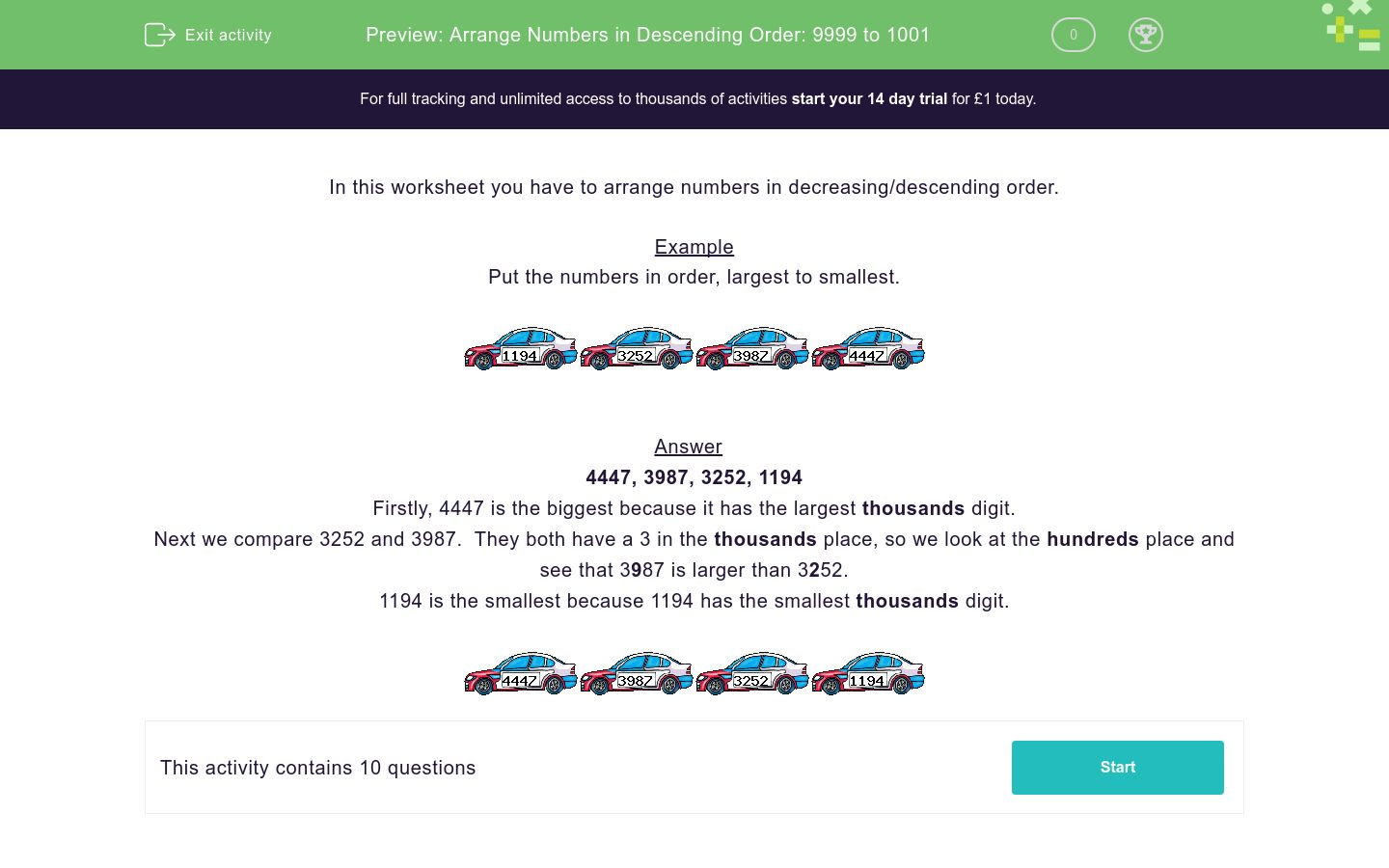Key stage:  KS 2

Curriculum topic:  Number: Number and Place Value

Curriculum subtopic:  Order/Compare Numbers Beyond 1000

Difficulty level:QUESTION 1 of 10

In this worksheet you have to arrange numbers  in decreasing/descending order

Example

Put the numbers in order, largest first.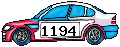Firstly, 4447 is the biggest because it has the largest thousands digit.

Next we compare 3252 and 3987.  They both have a 3 in the thousands place, so we look at the hundreds place and see that 3987 is larger than 3252.

1194 is the smallest because 1194 has the smallest thousands digit.Put the numbers in order, largest first.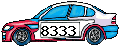2977 9968 6319 8333 1 2 3 4

Put the numbers in order, largest first.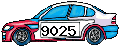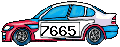9025 7665 5969 1566 1 2 3 4

Put the numbers in order, largest first.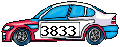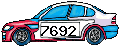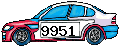3833 7692 9951 2823 1 2 3 4

Put the numbers in decreasing order.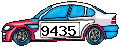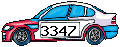9961 9435 3347 5715 1 2 3 4

Put the numbers in descending order.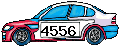9979 7622 7271 4556 1 2 3 4

Put the numbers in order, largest first.9663 6305 9240 9641 1 2 3 4

Put the numbers in order, largest first.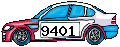7935 1535 9401 9448 1 2 3 4

Put the numbers in descending order.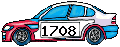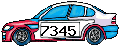2953 1708 7345 6744 1 2 3 4

Put the numbers in decreasing order.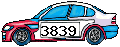3839 8290 5376 3685 1 2 3 4

Put the numbers in order, largest first.9453 5754 9084 5818 1 2 3 4
• Question 1

Put the numbers in order, largest first.2977 9968 6319 8333 1 2 3 4
• Question 2

Put the numbers in order, largest first.9025 7665 5969 1566 1 2 3 4
• Question 3

Put the numbers in order, largest first.3833 7692 9951 2823 1 2 3 4
• Question 4

Put the numbers in decreasing order.9961 9435 3347 5715 1 2 3 4
• Question 5

Put the numbers in descending order.9979 7622 7271 4556 1 2 3 4
• Question 6

Put the numbers in order, largest first.9663 6305 9240 9641 1 2 3 4
• Question 7

Put the numbers in order, largest first.7935 1535 9401 9448 1 2 3 4
• Question 8

Put the numbers in descending order.2953 1708 7345 6744 1 2 3 4
• Question 9

Put the numbers in decreasing order.3839 8290 5376 3685 1 2 3 4
• Question 10

Put the numbers in order, largest first.